Scrapbook - Zonohedra 16 images tree :: Geometry > Scrapbook - Zonohedra
In This Album:

 Image List Thumbnail Page
Zonohedra can be generated by taking a set of vectors (a star), calculating the displacements produced by all possible combinations of these vectors, and then calculating the convex hull of these displacements.

Polar zonohedra are produced from the star of the slanting edges of a right regular pyramid. Symmetric zonohedra can be produced by vectors taken from the edges and vertices of uniform polyhedra.

 Image List

Icosahedron all  Med    Lrg
 Description : Star is centre-to-vertex and edge vectors of an icosahedron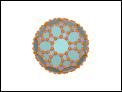Icosahedron edges  Med    Lrg
 Description : Star is edge vectors of an icosahedron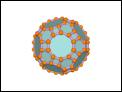Icosahedron vertices  Med    Lrg
 Description : Star is centre-to-vertex vectors of an icosahedronPolyhedron vertices  Med    Lrg
 Description : Star is centre-to-vertex vectors of a polyhedronPolyhedron vertices  Med    Lrg
 Description : Star is centre-to-vertex vectors of a polyhedronCube vertices  Med    Lrg
 Description : Rhombic dodecahedron. Star is centre-to-vertex vectors of a cubeCuboctahedron vertices  Med    Lrg
 Description : Truncated octahedron. Star is centre-to-vertex vectors of a cuboctahedron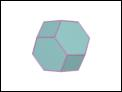Icosahedron vertices  Med    Lrg
 Description : Rhombic triacontahedron. Star is centre-to-vertex vectors of an icosahedronPolar Zonohedron 6  Med    Lrg
 Description : Polar zonohedron produced from a star of 6 vectors.Polar Zonohedron 7  Med    Lrg
 Description : Polar zonohedron produced from a star of 7 vectors.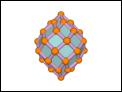Polar Zonohedron 10  Med    Lrg
 Description : Polar zonohedron produced from a star of 10 vectors and with each face a different colour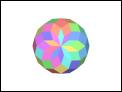Polar Zonohedron 20  Med    Lrg
 Description : Polar zonohedron produced from a star of 20 vectors and with a proper colouring of the faces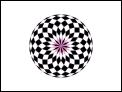Polar Zonohedron 100  Med    Lrg
 Description : Polar zonohedron produced from a star of 100 vectors.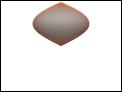Polar Zonohedron 100  Med    Lrg
 Description : Polar zonohedron produced from a star of 100 vectors.Polar Zonohedron 50  Med    Lrg
 Description : Polar zonohedron produced from a star of 50 vectors and with a proper colouring of the facesPolar Zonohedron 50  Med    Lrg
 Description : Polar zonohedron produced from a star of 50 vectors and with a proper colouring of the faces